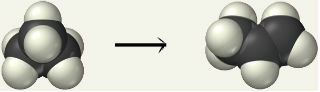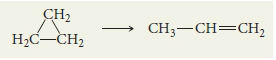# Problem: Cyclopropane rearranges to form propene in the gas phase.The reaction is first order in cyclopropane and has a measured rate constant of 3.36 x 10-5 s-1 at 720 K. If the initial cyclopropane concentration is 0.0505 M, what is the cyclopropane concentration after 251 minutes?

###### FREE Expert Solution

We are asked to determine the amount of the cyclopropane concentration after 251 minutes

The integrated rate law for a 1st-order reaction is as follows:

where rate= rate at time t, k = rate constant, t = time, initial rate = starting rate

94% (462 ratings)###### Problem Details

Cyclopropane rearranges to form propene in the gas phase.The reaction is first order in cyclopropane and has a measured rate constant of 3.36 x 10-5 s-1 at 720 K. If the initial cyclopropane concentration is 0.0505 M, what is the cyclopropane concentration after 251 minutes?

Frequently Asked Questions

What scientific concept do you need to know in order to solve this problem?

Our tutors have indicated that to solve this problem you will need to apply the Integrated Rate Law concept. You can view video lessons to learn Integrated Rate Law. Or if you need more Integrated Rate Law practice, you can also practice Integrated Rate Law practice problems.

What professor is this problem relevant for?

Based on our data, we think this problem is relevant for Professor Delgado's class at FIU.